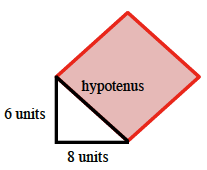### Home > GC > Chapter 2 > Lesson 2.3.2 > Problem2-109

2-109.

Draw a right triangle with legs of length $6$ and $8$ units, respectively, onto graph paper. Construct a square on the hypotenuse and use the square's area to find the length of the hypotenuse.

Draw the triangle and a square where one side is the hypotenuse.Count the number of unit squares contained within the square, giving each partial square a value of one-half.

Since the area of the square ($100$) is the length of the hypotenuse squared, the square root of the square's area is the length of the hypotenuse.

$\sqrt{100}$ = $10$